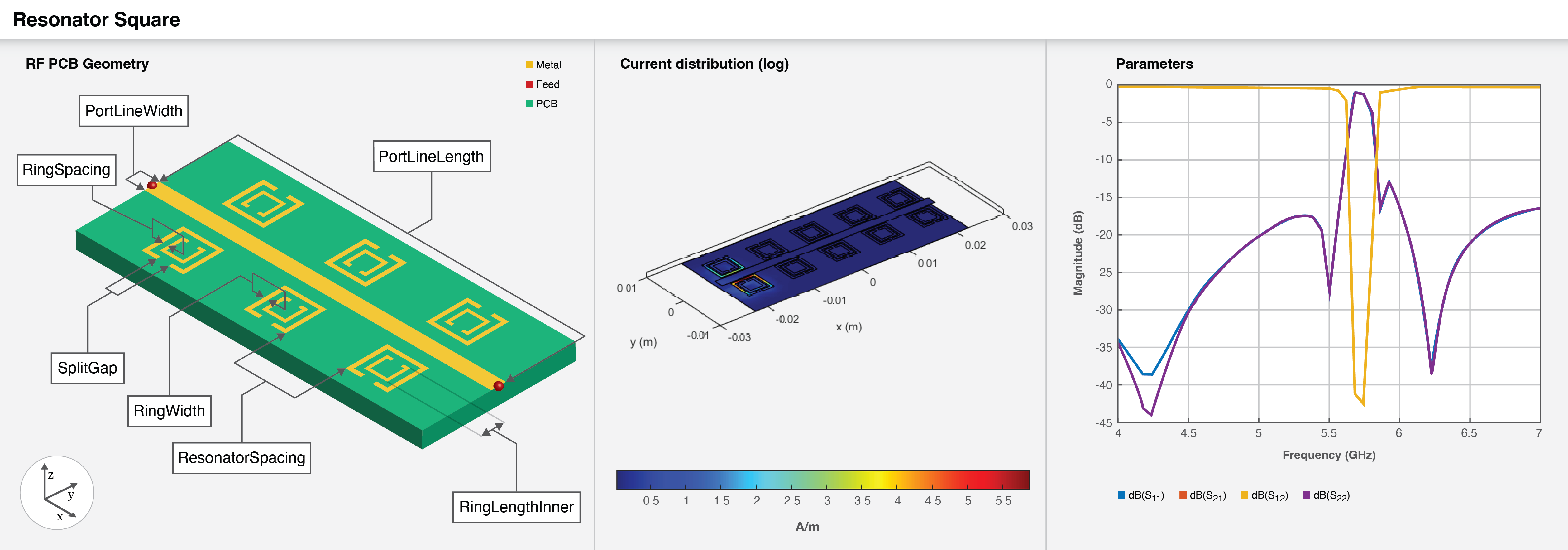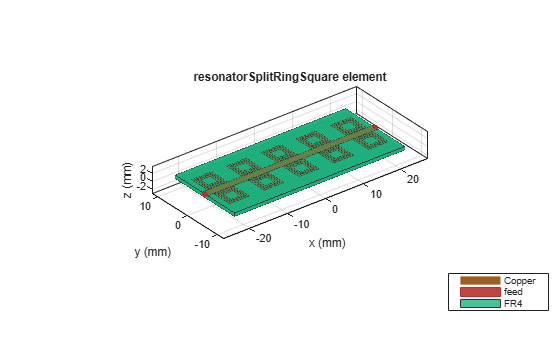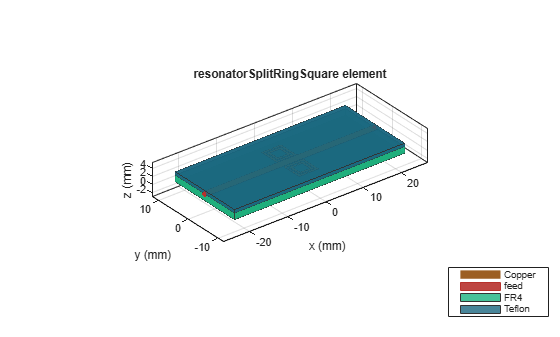# resonatorSplitRingSquare

Create microstrip line loaded with square split-ring resonator

Since R2022b

## Description

Use the `resonatorSplitRingSquare` object to create a microstrip line loaded with a square split-ring resonator.## Creation

### Syntax

``resonator = resonatorSplitRingSquare``
``resonator = resonatorSplitRingSquare(Name=Value)``

### Description

example

````resonator = resonatorSplitRingSquare` creates a microstrip line loaded with a square split-ring resonator on the X-Y plane. The default proprieties are for a design frequency of 5.7 GHz.```

example

````resonator = resonatorSplitRingSquare(Name=Value)` sets Properties using one or more name-value arguments. For example, `resonatorSplitRingSquare(RingWidth=0.045)` creates a square split-ring resonator with a ring width of `0.045` meters. Properties not specified retain their default values```

## Properties

expand all

Length of the inner ring of the ring resonator in meters, specified as a positive scalar.

Example: ```resonator = resonatorSplitRingSquare(RingLengthInner=0.045)```

Data Types: `double`

Width of the square ring in meters, specified as a positive scalar.

Example: ```resonator = resonatorSplitRingSquare(RingWidth=0.0009)```

Data Types: `double`

Spacing between the inner and outer square ring in meters, specified as a positive scalar.

Example: ```resonator = resonatorSplitRingSquare(RingSpacing=0.0008)```

Data Types: `double`

Split gap on the square ring in meters, specified as a positive scalar.

Example: ```resonator = resonatorSplitRingSquare(SplitGap=0.0008)```

Data Types: `double`

Gap between the resonator and the microstrip line in meters, specified as a positive scalar.

Example: ```resonator = resonatorSplitRingSquare(CouplingGap=0.00082)```

Data Types: `double`

Number of resonators on one side of the transmission line, specified as a positive scalar in the range [1,7]. The object supports a minimum of one resonator and a maximum of seven resonators.

Example: ```resonator = resonatorSplitRingSquare(NumResonator=4)```

Data Types: `double`

Spacing between the resonators when number of resonators is more than one in meters, specified as a positive scalar.

Example: ```resonator = resonatorSplitRingSquare(NumResonator=4)```

Data Types: `double`

Length of the microstrip line in meters, specified as a positive scalar.

Example: ```resonator = resonatorSplitRingSquare(PortLineLength=0.055)```

Data Types: `double`

Width of the microstrip line in meters, specified as a positive scalar.

Example: ```resonator = resonatorSplitRingSquare(PortLineWidth=0.0061)```

Data Types: `double`

Height of the resonator from the ground plane in meters, specified as a positive scalar.

Example: ```resonator = resonatorSplitRingSquare(Height=0.020)```

Data Types: `double`

Width of the ground plane in meters, specified as a positive scalar.

Example: ```resonator = resonatorSplitRingSquare(GroundPlaneWidth=0.2241)```

Data Types: `double`

Type of dielectric material to use as a substrate, specified as a `dielectric` object. The thickness of the default dielectric material has the following parameters: `(Name={'FR4'},EpsilonR=3.38,LossTangent=0.0002,Thickness=0.813e-3)`.

Example: `d = dielectric("FR4");` `resonator = resonatorSplitRingSquare(Substrate=d)`

Type of metal to use in the conducting layers, specified as a `metal` object.

Example: `m = metal("PEC");` `resonator = resonatorSplitRingSquare(Conductor=m)`

## Object Functions

 `charge` Calculate and plot charge distribution `current` Calculate and plot current distribution `design` Design hairpin filter around specified frequency `feedCurrent` Calculate current at feed port `getZ0` Calculate characteristic impedance of transmission line `layout` Plot all metal layers and board shape `mesh` Change and view mesh properties of metal or dielectric in PCB component `shapes` Extract all metal layer shapes of PCB component `show` Display PCB component structure or PCB shape `sparameters` Calculate S-parameters for RF PCB objects

## Examples

collapse all

Create a default square split-ring resonator.

`resonator = resonatorSplitRingSquare`
```resonator = resonatorSplitRingSquare with properties: RingLengthInner: 0.0036 RingWidth: 5.0000e-04 RingSpacing: 3.0000e-04 SplitGap: 5.0000e-04 CouplingGap: 2.5000e-04 NumResonator: 5 ResonatorSpacing: 0.0040 PortLineLength: 0.0450 PortLineWidth: 0.0019 Height: 8.1300e-04 GroundPlaneWidth: 0.0200 Substrate: [1x1 dielectric] Conductor: [1x1 metal] ```

View the resonator.

`show(resonator)`Create a split-ring square with one resonator.

```resonator = resonatorSplitRingSquare(NumResonator=1); resonator.Height = 0.0016;```

Create a dielectric object with two dielectrics. Use this substrate in the resonator.

```sub = dielectric('FR4','Teflon'); sub.Thickness =[0.0016 0.0008]; resonator.Substrate = sub;```

View the resonator.

```figure; show(resonator);```Pozar, David.M. Microwave Engineering Singapore; JohnWiley and Sons. Inc, 2012.

 Mahyuddin, Muzlifah and Nur Farah Syazwani Ab. Kadir. Design of a 5.8 GHz Bandstop Filter Using Split Ring Resonator Array

 Mahyuddin, Muzlifah and Nur Farah Syazwani Ab. Kadir. “A 10 GHz Low Phase Noise Split-Ring Resonator Oscillator.” International Journal of Information and Electronics Engineering, 2013.